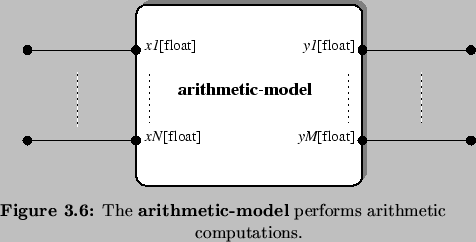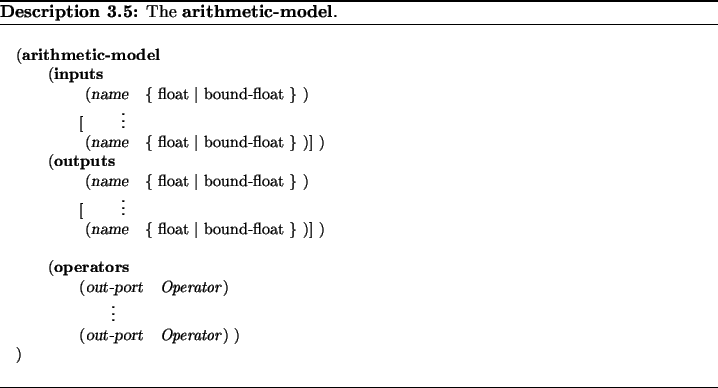Next: 3.6.2 The Curve-Calculator-Model Up: 3.6 Auxiliary Models Previous: 3.6 Auxiliary Models

## 3.6.1 The Arithmetic-Model

The arithmetic-model as shown in Figure 3.6 is a general purpose model which is used to perform arithmetic operations on the data presented at its input ports. It will usually be used in conjunction with the network-model. An arithmetic operator which computes the results for that output port has to be defined for each output port. Each operator may use the values of all input ports to compute its value. These operators are expressed in a LISP prefix notation. Input ports as well as output ports can either be of type float or bound-float as shown in Description 3.5. Example 3.3 depicts an arithmetic-model which computes the difference between the float values at its input ports vt and vt-nom, and delivers it at the output port vt-mismatch.Rudi Strasser
1999-05-27